CAT  >  Sample IIFT Quantitative Ability Quiz

# Sample IIFT Quantitative Ability Quiz

Test Description

## 22 Questions MCQ Test IIFT Mock Test Series | Sample IIFT Quantitative Ability Quiz

Sample IIFT Quantitative Ability Quiz for CAT 2022 is part of IIFT Mock Test Series preparation. The Sample IIFT Quantitative Ability Quiz questions and answers have been prepared according to the CAT exam syllabus.The Sample IIFT Quantitative Ability Quiz MCQs are made for CAT 2022 Exam. Find important definitions, questions, notes, meanings, examples, exercises, MCQs and online tests for Sample IIFT Quantitative Ability Quiz below.
Solutions of Sample IIFT Quantitative Ability Quiz questions in English are available as part of our IIFT Mock Test Series for CAT & Sample IIFT Quantitative Ability Quiz solutions in Hindi for IIFT Mock Test Series course. Download more important topics, notes, lectures and mock test series for CAT Exam by signing up for free. Attempt Sample IIFT Quantitative Ability Quiz | 22 questions in 20 minutes | Mock test for CAT preparation | Free important questions MCQ to study IIFT Mock Test Series for CAT Exam | Download free PDF with solutions
 1 Crore+ students have signed up on EduRev. Have you?
Sample IIFT Quantitative Ability Quiz - Question 1

### In a school 6 class hours are to be managed with 5 different subjects. What is the total number of ways in which this can be done if each subject hour is to be conducted at least once?

Detailed Solution for Sample IIFT Quantitative Ability Quiz - Question 1

In the available 6 slots, 5 subjects can be organized in 6P5 = 720 ways.
The remaining one slot can be filled with any one of the 5 subjects.
Hence total number of ways = 720 x 5 But since one subject is repeated, the total number of distinct possibilities =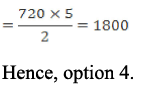Sample IIFT Quantitative Ability Quiz - Question 2

### If the natural numbers x and y satisfy the equation x2 - xy - 101 = 0, then which of the following is a possible value of (x + y)?

Detailed Solution for Sample IIFT Quantitative Ability Quiz - Question 2

We simplify the equation x2 - xy - 101 = 0 as, x(x- y) = 101 = 1 * 101. As x and y are integers, we have, x = 1 and x - y = 101, or x = 101 and x - y = 1.
If x  = 1 , y = 1 - 101 = -100.
If x = 101 , y = 101 - 1 = 100.

As x and y are natural numbers, we must disregard the solution (x, y) = (1, - 100).
There is only one solution: x = 101 and y = 100.
For this set, we have (x + y) = 101 + 100 = 201.
Hence, option 4.

Sample IIFT Quantitative Ability Quiz - Question 3

### For starting an import export business, three friends Amav, Anubha and Astha contributed various amounts for different time periods. Amav contributed a sum of Rs. 1,00,000 from the first day of January and withdrew the same in April at the end. Anubha invested Rs. 50,000 in April and withdrew the same after 6 months. Astha invested Rs. 60,000 for 7 months. At the end of the year the net profit was 14,000. The three decided to keep aside 20% of the profit for business development and the rest is to be divided among them. What will the profit share of Anubha?

Detailed Solution for Sample IIFT Quantitative Ability Quiz - Question 3

Ratio of the shares of Amav, Anubha and Astha = 1,00,000 x 4 : 50,000 x 6 : 60,000 x 7 = 20: 15 : 21
Profit to be divided = 80% of Rs. 14,000 = Rs. 11,200.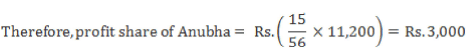Hence, option 2.

Sample IIFT Quantitative Ability Quiz - Question 4

If log (4x + 1) + 2x = log 15 + x log 4, which of the following is tme? (Assume that all logarithms are to the base 10.)

Detailed Solution for Sample IIFT Quantitative Ability Quiz - Question 4

log (4x + 1) + 2x = log 15 + x log 4

Therefore log (4x + 1) = log 15 + log 4x - 2x.

log (4x + 1) - log 4x = log 15 - 2x log 10 ...(since log1010 = 1)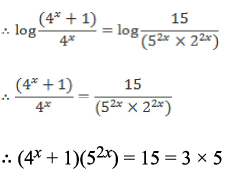We try to equate each factor on the L.H.S. to a corresponding factor on the R.H.S. and find that, for x = 1/2, we have (4x + 1) = 3 and (5)2x = 5 Thus, x = 1/2. For this value of x, we have 2x = 1, 4x = 2 and 8x = 4.
Only 4x = 2 is a prime.
Hence, option 3.

Sample IIFT Quantitative Ability Quiz - Question 5

An IT company CEO tells the truth 3/5th of the time, and 1/4th of the total BPO’s are going to close down in the next month. If four IT company CEOs say that a particular BPO is going to close down in the next month, then what is the probability that the particular BPO is indeed going to close down in the next month?

Detailed Solution for Sample IIFT Quantitative Ability Quiz - Question 5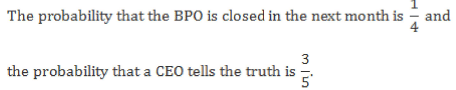The number of outcomes that the BPO would close down is given by: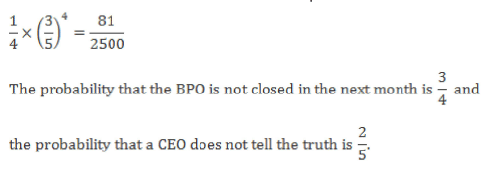The number of outcomes that the BPO would close down is given by: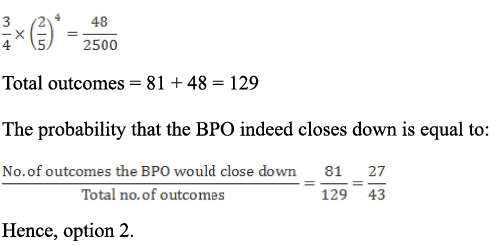Sample IIFT Quantitative Ability Quiz - Question 6

Consider a triangle and cyclic rectangle of area A. If there is a threefold increase in all the sides of the rectangle as well as the triangle, then the area of the triangle is

Detailed Solution for Sample IIFT Quantitative Ability Quiz - Question 6

Consider a rectangle of length l cm and breadth b cm.  Its area = A = l x b.

A threefold increase in its sides will make the new length equal to 3l and the new breadth equal to 3b. New area of the rectangle = 9(l x b) = 9A. Similarly, consider a triangle of sides a cm, b cm and c cm.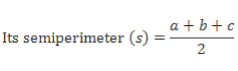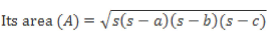A threefold increase in its sides will make the new sides equal to 3a, 3b and 3c.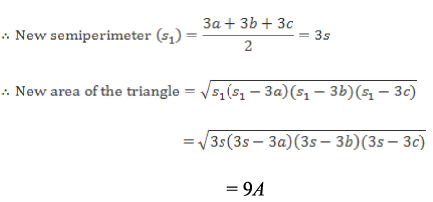Area of the triangle will be equal to the area of the rectangle. Hence, option 4.

Sample IIFT Quantitative Ability Quiz - Question 7

There are some goods in a shop. If one-fifth of these goods are sold at a 10% loss and 20% of the remaining goods are sold at “no profit no loss”, then at what profit percentage should the remaining goods be sold so as to obtain 25% profit on the entire transaction? Assume that the goods have a uniform cost i.e. each item costs the same.

Detailed Solution for Sample IIFT Quantitative Ability Quiz - Question 7

Let there be 100 items, each costing Re. 1.
Thus, total cost price = Rs. 100.
For an overall profit percentage of 25%, total selling price = 100 x 1.25 = Rs. 125
Since one-fifth of the goods are sold at a 10% loss, it means that 20 items are sold at Rs. 0.9 each.
So, amount received from this sale = 20 x 0.9 = Rs. 18. 20% of the remaining goods are sold at neither profit nor loss. Hence, 0.2 x 80 i.e 16 items are sold at Re. 1 each.

So, amount received from this sale = 16 x 1= R s . 16 Now, there are 100 - (20 + 16) = 64 items left to be sold.
Hence, amount that should be received from these 64 goods = 125 - (18 + 16) = Rs. 91.

Hence, each of these items is sold at Rs. 91/64.

Profit percentage = {[(91/64) - 1]/1} x 100 = 42.19% Hence option 3.

Sample IIFT Quantitative Ability Quiz - Question 8

A bucket is kept inside a bucket, which in turn is kept inside the other bucket, which is again kept inside another bucket and so on. This process is repeated infinite times. All the buckets have a leak such that water leaks from any bucket to the bucket below it. The rate of leakage in each bucket is half of the rate at which the bucket is filled. What is the total amount of water leaking at any point of time if the water gets filled in the uppermost bucket at a rate of 2x?

Detailed Solution for Sample IIFT Quantitative Ability Quiz - Question 8

As the first bucket is filled at a rate of 2x, water leaks from the first bucket at a rate of x.
The second bucket is filled at a rate of x, therefore, water leaks from this bucket at a rate of 0.5x.
The third bucket is filled at a rate of 0.5x, therefore, water leaks from this bucket at a rate of 0.25x

Continuing in this manner we get an infinite geometric progression with a = x and r = 1/2

So, sum of the amount of water leaking at any point of time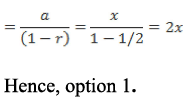Sample IIFT Quantitative Ability Quiz - Question 9

In a certain village, 25% of the total number of villagers own a phone and 15% of the total number of villagers own a car. There are 2000 villagers who own both - a car as well as a phone. If 65% of the total number of villagers own neither a car nor a phone, then how many villagers own only a phone?

Detailed Solution for Sample IIFT Quantitative Ability Quiz - Question 9

Since 65% of the villagers own neither a car nor a phone, 35% of the villagers own atleast one of the two.
Let the percentage of villagers who own a car as well as a phone be x%. 25% of the villagers own a phone and 15% of the villagers own a car. (25 - x) + x + (15 - x) = 35. So  x = 5
Hence, 25 - 5 i.e. 20% of the villagers own only a phone.

Since 2000 villagers own both a phone and a car, 5% of the total number of villagers corresponds to 2000.
Hence, the number of villagers who own only a phone (20%) is 4 * 2000 = 8000
Hence, option 3.

Sample IIFT Quantitative Ability Quiz - Question 10

What are the total number of ways in which 10 boys can be seated in a row if two particular boys must always have exactly 3 boys in between them?

Detailed Solution for Sample IIFT Quantitative Ability Quiz - Question 10

Let the two particular boys be A and B.
They can be arranged in 2 ways after keeping a gap of 3 seats between them.
Three boys who are to be seated in between A and B can be selected from remaining 8 boys and arranged in 8P3 ways.
The remaining 5 boys can be arranged in 5! ways.
The group of 5 boys including A and B and the ones sitting between them can be seated in the arrangement in 6 ways.
The total number of ways in which all the 10 boys can be seated is 2 x 8P3 x 5! x 6 = 8P3 x 6! x 2 = 8P3 x 1440 Hence, option 2.

Sample IIFT Quantitative Ability Quiz - Question 11

Two bananas, three cakes, four colas, five books cost Rs. 59.5. Two bananas, four cakes, five colas cost Rs. 39.5. If the difference between the cost of the book and the cost of the cake is Rs. 2 and one of the two costs is some rupees and 50 paisa then find the cost of the cola. (Assume that none of them come free of cost, the cost of any article cannot be greater than 7 and the cost of any book is greater than the cost of cola).

Detailed Solution for Sample IIFT Quantitative Ability Quiz - Question 11

Let the cost of the banana, cake, cola and book be b, c, a, d respectively.
So,
2b + 3c + 4a + 5d= 59.5 ... (i)

2b + 4c + 5a = 39.5 ... (ii)

Subtracting (i) by (ii) we get,

- c - a + 5d = 20 ... (iii)

We are given that, d - c = 2 ... (iv) And d or c has 50 paisa in it.
So we check all the combination where the difference between the cake and the book.

Also as none of them come free, the cost of anything cannot be greater than 7 and the cost of book is greater than the cost of cake, so using equation (iv) the combinations of the cost of a book and a cake are (2.5, 0.5), (3.5, 1.5), (4.5, 2.5), (5.5, 3.5), (6.5, 4.5).
By substituting each combination of the book and the cake in equation in (iii) we get, the value of the book and the cake to be (5.5, 3.5) and the corresponding value of the cola is Rs. 4 Hence, option 4.

Sample IIFT Quantitative Ability Quiz - Question 12

The selling price of fuel is Rs. 66 and the petrol pump has a gain of 10%. However due to inflation, the selling price increased to Rs. 75. What is the gain percentage if the cost price remains the same?

Detailed Solution for Sample IIFT Quantitative Ability Quiz - Question 12

The petrol pump has a gain of 10%. i.e., if CP = Rs. 100, SP = Rs. 110. It is given that SP = Rs. 66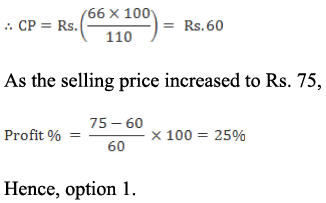Sample IIFT Quantitative Ability Quiz - Question 13

A vendor has ten 20 litre bottles. He fills bottle 1 with 20 litres of petrol from a barrel containing 120 litres of pure petrol. He then adds 20 litres of kerosene to the barrel. He then fills bottle 2 with 20 litres of the mixture from the barrel and adds 20 litres of kerosene to the barrel. The process is repeated until all the 10 bottles are filled. What is the amount of kerosene in the 5th bottle?

Detailed Solution for Sample IIFT Quantitative Ability Quiz - Question 13

Petrol in 1st bottle = 20 litres.

Petrol in 2nd bottle = 20 x (100/120) = 20 x (5/6) litres.

Petrol in 3rd bottle = 20 x (5/6)2 litres.

Petrol in 5th bottle = 20 x (5/6)4 = 3125/324 litres.

Kerosene in the 5th bottle = 20 - (3125/324) = 3355/324 litres Hence, option 2.

Sample IIFT Quantitative Ability Quiz - Question 14

Prof. Ajit wrote a number 419 on the blackboard and asked Sheetal, Komal and Manju to convert it into a base system of their choice. Sheetal wrote the number as 643 while Manju wrote it as 12203. If Komal’s base system was the average of Sheetal and Manju’s base system; and Sheetal’s base system was twice Maniu’s base system, what number did Komal write?

Detailed Solution for Sample IIFT Quantitative Ability Quiz - Question 14

Since the largest digit in Sheetal’s number is 6, her base system has to be atleast 8. It has to be an even number as it is twice the number system that Manju used.
Similarly, Manju’s base system has to be atleast 4.
If Sheetal wrote the number in base system x,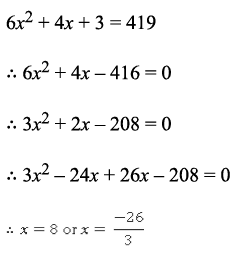Thus, Sheetal wrote her number in base 8 while Manju wrote it in base 4.
Since Komal’s base system was the average of these two, Komal wrote her number in the base system 6.
Hence, option 3 can be directly eliminated as 6 cannot be a digit in base system 6.

Now, consider options 1 and 2; and check if they give 419.

Option 1: 2423

2(6)3 + 4(6)2 + 2(6)1 + 3 = 2(216) + 4(36) + 12 + 3 = (591)10
Hence, option 1 can be eliminated.

Option 2: 1535

1(6)3 + 5(6)2 + 3(6)1 + 5 = 216 + 180+ 18 + 5 = (419)10
Hence, option 2.

Sample IIFT Quantitative Ability Quiz - Question 15

If the roots of the equation ax2 + bx + c = 0 are in the ratio p : q, then which of the following is true?

Detailed Solution for Sample IIFT Quantitative Ability Quiz - Question 15

Consider the given equation,

ax2 + bx + c = 0
Since roots are in the ratio p : q, let the roots be pk and qk.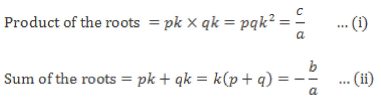On substituting the value of k from equation (ii) to equation (i), we get, b2pq = ac(p + q)2
Hence, option 2.

Sample IIFT Quantitative Ability Quiz - Question 16

Hari, Gopi and Sufi enter into a partnership. Gopi contributes one-fourth of the whole capital while Hari contributes twice as much as Gopi and Sufi together contribute. If the profit at the end of the year is Rs. 4800, how much would each receive?

Detailed Solution for Sample IIFT Quantitative Ability Quiz - Question 16

Let the total capital be 12x.
Then Gopi contributes 3x.
Hari contributes twice as much as Gopi and Sufi together contribute. If Sufi contributes y, then Hari contributes 2(3x + y). Therefore 3x + y + 2(3x+ y) = 12x.

y = x.

Sufi contributes x and Hari contributes 8x.
Gopi’s, Hari’s and Sufi’s contributions are in the ratio 3 : 8 : 1. Their profits also would be in the same proportion and would be Rs. 1200, Rs. 3200 and Rs. 400.
Hence, option 2.

Sample IIFT Quantitative Ability Quiz - Question 17

A circular disc of radius 5 cm and negligible width is painted black on both the side. A cuboid of width 5cm, length 10cm and of height 5 cm is pasted lengthwise symmetrically over one of its diameters. The remaining part of the disc which is not pasted to the cuboid is removed. What is the area of the disc pasted on the cuboid?

Detailed Solution for Sample IIFT Quantitative Ability Quiz - Question 17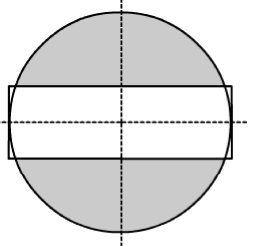The rectangular cube is pasted lengthwise symmetrically over the diameter.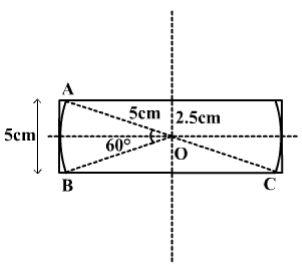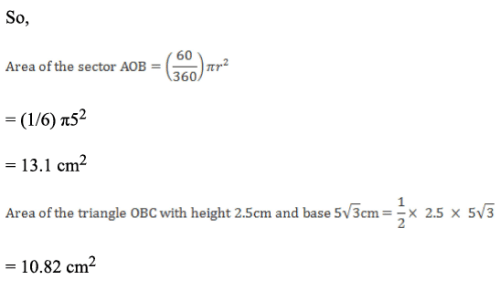Area of the disc attached to the rectangular cube = 2 x (area of the sector + area of the triangle) = 2 x (13.1+ 10.82) = 47.84 cm2 Hence, option 4.

Sample IIFT Quantitative Ability Quiz - Question 18

If n is an integer, and k = 0.010101010101 x 10n. If k is greater than 1000, but less than 10,00,000 then what is the sum of all possible values of n?

Detailed Solution for Sample IIFT Quantitative Ability Quiz - Question 18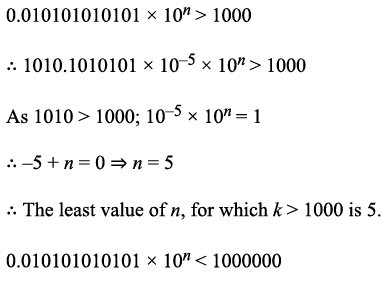101010.10101 x 10-7 x 10n < 1000000 < 1010101.0101 x 10-8 x 10n. Therefore
-7 + n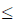0.  n7. Thus, for 1000 < k < 1000000; n = 5, 6, 7. The sum of possible values of n = 5 + 6 + 7 = 18. Hence, option 3.

Sample IIFT Quantitative Ability Quiz - Question 19

Three brothers make one statement each:

Ravi - I have made a rhombus having an area of 24 sq.cm.
Raju - I have made a rectangle using the diagonals of Ravi’s rhombus.
Ronak - The area of my square is thrice the area of Raju’s rectangle.

What is the side of Ronak’s square?

Detailed Solution for Sample IIFT Quantitative Ability Quiz - Question 19

Let x and y be the length of diagonals of Ravi’s rhombus.  Area of rhombus = 24 = (product of diagonals)/2 = xy/2. xy = 48 Now, x and y also correspond to the sides of Raju’s rectangle.
Area of rectangle = xy = 48 sq.cm. Area of Ronak’s square = 48(3) = 144 sq.cm. Length of side of square = 12 cm Hence, option 2.

Sample IIFT Quantitative Ability Quiz - Question 20

A particular coaching centre for IIFT entrance decides to hold its mock exam in the form of a CBT. The questions for the exam are taken from a large database which consists of questions of five difficulty levels: Very Easy (VE), Easy (E), Medium (M), Difficult (D) and Very Difficult (VD). The probability of a question from one of these levels is 0.1, 0.2, 0.4, 0.2 and 0.1 respectively and the probability of a particular student answering the question from one of these levels is 90%, 80%, 70%, 50% and 20% respectively. What is the probability of a student answering a random question from this database incorrectly?

Detailed Solution for Sample IIFT Quantitative Ability Quiz - Question 20

The following table lists the different probabilities: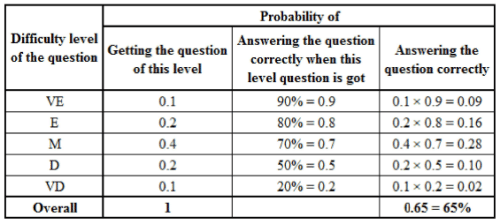From, the above table, it can be seen that the probability of answering the random question correctly is 0.65. The probability of answering the random question incorrectly = 1 - 0.65 = 0.35
Hence, option 4.

Sample IIFT Quantitative Ability Quiz - Question 21

Anand and Shiva can complete a piece of work in 18 days and 22 days respectively. They begin the work together, but Shiva leaves after some days and Anand finishes the remaining work in 14 days. After how many days did Shiva leave?

Detailed Solution for Sample IIFT Quantitative Ability Quiz - Question 21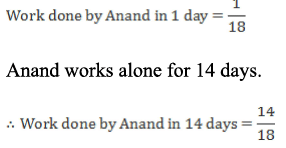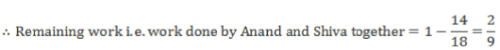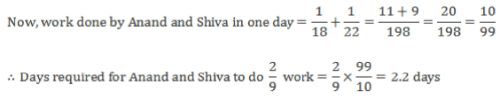i.e. Shiva left after 2.2 days.
Hence, option 2.

Sample IIFT Quantitative Ability Quiz - Question 22

NORSK is involved in Software, Hardware and Technical support for International Business Machines Corporation. Current years Hardware sales of 116.8 million dollars is expected to achieve a sales growth of 7.25%; Software sales is expected to grow by 8.2% and reach 59.3 million dollars next year; sales through technical support of 70.4 million dollars is expected to increase sales by 9.99%. What is the NORSK expected sales growth for the next year?

Detailed Solution for Sample IIFT Quantitative Ability Quiz - Question 22

Total sales this year = 116.8 + (59.3 x 100)/108.2 + 70.4 ~ 242 million dollars
Expected sales next year = (116.8 x 1.0725) + 59.3 + (70.4 x 1.0999) ~ 262 million dollars.

Expected sales growth: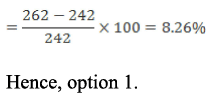## IIFT Mock Test Series

1 docs|46 tests
 Use Code STAYHOME200 and get INR 200 additional OFF Use Coupon Code
Information about Sample IIFT Quantitative Ability Quiz Page
In this test you can find the Exam questions for Sample IIFT Quantitative Ability Quiz solved & explained in the simplest way possible. Besides giving Questions and answers for Sample IIFT Quantitative Ability Quiz, EduRev gives you an ample number of Online tests for practice

## IIFT Mock Test Series

1 docs|46 tests

### How to Prepare for CAT

Read our guide to prepare for CAT which is created by Toppers & the best Teachers